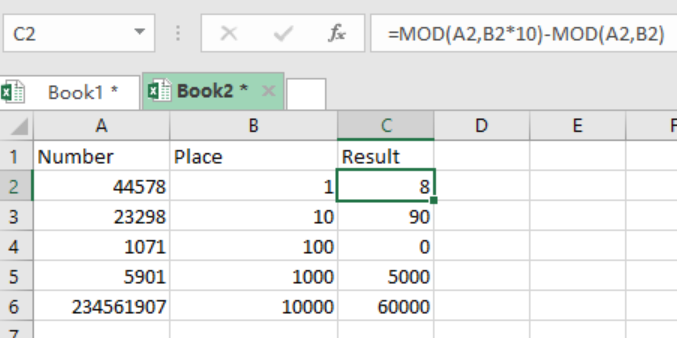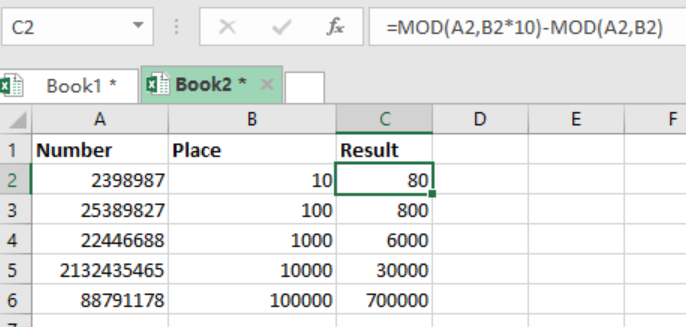Get instant live expert help with Excel or Google Sheets“My Excelchat expert helped me in less than 20 minutes, saving me what would have been 5 hours of work!”

#### Post your problem and you’ll get Expert help in seconds.

Your message must be at least 40 characters
Our professional Expert are available now. Your privacy is guaranteed.

# Get Number at Place Value

We can use a formula that is based on MOD to get a number at place value. Place value in this context refers to ones, tens, hundredths and so on. This post provides a step-by-step guide on how to get a number at a specified place value.Figure 1: Get number at place value

## Syntax of the formula

`=MOD(number, place*10) – MOD(number, place)`

## Understanding how the formula works

This formula works by subtracting one MOD result from another. In the formula, the MOD function takes a number and a divisor, and then returns the remainder after carrying out the division.

In the example below, we have the formula solved as below;

`=MOD(A2,B2*10) – MOD(A2,B2)`

`=MOD(44578, 1*10) – MOD(44578,1)`

`=8-0`

`=8`

## ExampleFigure 2: Example of MOD function

Explanation: We use the number with the divisor which is equals the place value we want 10 times in the first MOD.  However, when it comes to the second MOD, we only use the number with the divisor which is equal to the place value we are seeking.

## Instant Connection to an Expert through our Excelchat Service

Most of the time, the problem you will need to solve will be more complex than a simple application of a formula or function. If you want to save hours of research and frustration, try our live Excelchat service! Our Excel Experts are available 24/7 to answer any Excel question you may have. We guarantee a connection within 30 seconds and a customized solution within 20 minutes.

### Did this post not answer your question? Get a solution from connecting with the expert.Another blog reader asked this question today on Excelchat:
Solution examplesI need help with this formula. I need this to include months =INT((M4)/7)&" weeks"&IF(MOD(M4,7)=0,"",", "&INT(MOD(M4,7))&" days") I have the number of Days to Start with
Solved by F. A. in 29 minsneed help with the different sheets and formula IF(A4=B4,0,NETWORKDAYS(A4+1,B4,\$E\$9:\$E\$42) +0.5*(INT((+B4-A4+1)/7)+IF(WEEKDAY(A4+1)+MOD (B4-A4-1,7)>=7,1,0)))
Solved by O. D. in 11 minsi have this formula currently which works but not if I want it for every day - any ideas ?? (NETWORKDAYS.INTL(L1362,M1362,11,BH!A:A)-1)*("17:30"-"8:30")+IF(NETWORKDAYS.INTL(M1362,M1362,11,BH!A:A),MEDIAN(MOD(M1362,1),"8:30","17:30"),"17:30")-MEDIAN(NETWORKDAYS.INTL(L1362,L1362,11,BH!A:A)*MOD(L1362,1),"8:30","17:30")
Solved by A. D. in 28 mins## Subscribe to Excelchat.coAnother blog reader asked this question today on Excelchat: